# Effects of elbow structure of natural gas pipeline on condensation of water vapor

Effects of elbow structure of natural gas pipeline on condensation of water vapor

Xueqi Gao*Yuhou Zhu Jianjun Wang Youhai Jin

Multiphase Flow Separation Engineering Technology Center, China University of Petroleum, Qingdao 266580, China

SINOPEC Research Institute of Safety Engineering, Qingdao 266104, China

Corresponding Author Email:
gxqi2003@163.com
Page:
626-632
|
DOI:
https://doi.org/10.18280/ijht.350320
|
Accepted:
|
Published:
30 September 2017
| Citation

OPEN ACCESS

Abstract:

Condensation characteristics of gas pipelines containing little vapor in different elbows have been studied using a numerical calculation method. This is for the purpose of addressing the problem regarding condensed water corroding the inner wall of pipelines. Based on the condensation model of pipelines with low vapor content, the water vapor condensation process in a natural gas pipeline is simulated by UDF within Fluent software. Pipeline pressure simulated by Fluent is compared with the pressure calculated using the Friedel method. The error is less than 10%, which verifies the accuracy of the numerical simulation results. By analyzing non-uniformity distribution of the flow field caused by secondary circulation in different elbows, the simulation model was established to search for distribution of water vapor condensation in pipelines. The results show that the condensate distributions of water vapor in the elbow are non-uniform and mainly focus on the downstream of the inner elbow. Under the same conditions of flow rate and temperature difference of the inner and outer wall, condensation distribution in liquid phase on the inside elbow was compared at four different elbow angles such as 15°, 30°, 45° and 60°. The results indicate that maximum and minimum condensed vapor are separately generated at 15° and 60° elbow which can provide theoretical references for reducing the corrosion of natural gas pipelines.

Keywords:

elbow, condensate, two-phase flow, UDF

1. Introduction

With the increase in population and improvement of living standards, human demand for energy has increased dramatically. Natural gas is widely used in many fields because of its abundance and cleanliness. At present, natural gas is mainly transported by pipelines. In the transport process of wet natural gas or incomplete dehydrated natural gas, a gas-liquid phase flow system does exist in pipelines. Liquid film and droplets generated by condensed water, provide electrolytes for electrochemical corrosion. This causes further corrosion which can lead to oil and gas leakage, subsequently harming the environment. The study of gas-liquid two-phase flow in natural gas pipelines is of great significance when it comes to preventing and controlling pipeline corrosion.

In recent years, a significant amount of domestic and foreign research has been carried out on gas-liquid two-phase flow in natural gas pipelines  . The pattern of gas-liquid phase flow in horizontal pipes was studied with numerical simulation and experiments by Swanand M.Bhagwat et al. . Its simulation results were in agreement with the experimental results. Xiaorui Guan et al.  studied the wavy-film flow and droplet transport in a high-pressure natural gas pipeline, under low liquid loading through a hybrid model and an Eulerian wallfilm model. They found that the film in axial direction in the straight pipeline fluctuated periodically. The flow at the corner increased and the droplets were transferred to the upper part of the pipe to form a liquid film. Van’t Westende et al.  investigated the influence of vortex flow on droplet behaviors through large eddy simulation (LES). They concluded that the presence of second rate increased the presence of central liquid droplets in the pipeline. Weidong Li et al.  studied the distribution law of the transient liquid film around the pipeline through the experimental method of the two-parallel conductance probe. They proposed a mathematical model for prediction of the circumferential distribution of liquid film thickness. Fachun Liang et al.  theoretically studied the distribution characteristics of horizontal annular liquid film flow. They found that the axial distribution of liquid film was uneven. The liquid film was thickest at the bottom but lessened when it deviated from it. Hao Hou et al.  studied the gas-liquid two-phase flow in horizontal pipelines through the conductance method. They concluded that the thickness of liquid film outside the pipeline decreased with the increase of pipe spacing, while the fluctuation of liquid film increased. Andrea et al.  proposed that the degree of asymmetry in the annular liquid film could be predicted by analyzing the dimensionless number. Yuxing Li et al.  investigated the formation mechanism and distribution of internal liquid film in natural gas. They concluded that the formation of liquid film in annular flow was due to the interfacial unstable wave in stratified flow, which increased over time. Banafi et al  found that the pressure gradient of gas-liquid two-phase flow under low hydraulic pressure, can be predicted better by the Hamersma-Hart  model.

Scholars focus on the manifold flow pattern and influencing factors of gas-liquid two-phase flow in natural gas pipelines, few of them pay attention to the condensation characteristics of water vapor in natural gas pipelines. We have established a condensation model embedded in CFD calculation software and analyzed the reasons for the changes of condensate in the pipe, based on the characteristics of low vapor content in natural gas pipelines. In the present study, the distribution of condensate in the pipeline is monitored and the influence of the pipeline characteristics on the condensation of water vapor is analyzed. The results of the present study provide a reference for anticorrosion of natural gas pipeline.

2. Numeral Schemes

2.1 Parameter settings

Elbow diagrams are shown in Fig.1. The inner diameter of the pipe is 70 mm, the elbow angles are 15 °, 30 °, 45 ° and 60 ° respectively, the radius of elbow is 105mm and the total lengths of the pipes with different angles are 1500 mm. Based on the results of grid independence tests, the grid size is set to 2.7 mm.

## 1.png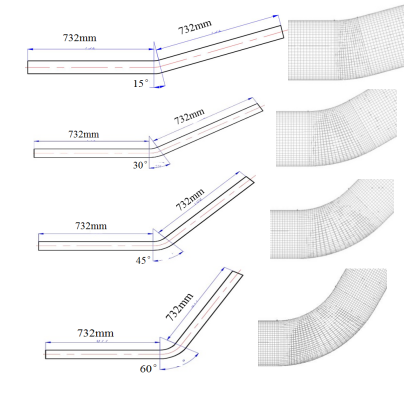Figure 1. Pipeline geometry model

2.2 Condensation model settings

For the gas phase in this paper, the fluid flow is governed by control equations within FLUENT software. These include: continuity equation, momentum equation and energy equation as shown in Eqs. (1–3).

The continuity equation is described as:

$\frac{\partial \rho_{\mathrm{g}}}{\partial t}+\frac{\partial}{\partial x_{\mathrm{j}}}\left(\rho_{\mathrm{g}} u_{\mathrm{j}}\right)=S_{\mathrm{m}}$     (1)

The momentum equation is described as:

$\frac{\partial}{\partial t}\left(\rho_{\mathfrak{g}} u_{\mathrm{i}}\right)+\frac{\partial}{\partial x_{\mathrm{j}}}\left(\rho_{\mathrm{g}} u_{\mathrm{j}} u_{\mathrm{i}}\right)=-\frac{\partial p}{\partial x_{\mathrm{i}}}$

$+\frac{\partial}{\partial x_{\mathrm{j}}}\left[\mu\left(\frac{\partial u_{\mathrm{j}}}{\partial x_{\mathrm{i}}}+\frac{\partial u_{\mathrm{i}}}{\partial x_{\mathrm{j}}}-\frac{2}{3} \frac{\partial u_{\mathrm{j}}}{\partial x_{\mathrm{j}}}\right)\right]+\Delta \rho_{\mathrm{g}} g_{\mathrm{i}}+S_{\mathrm{u}}$     (2)

The energy equation is described as:

$\frac{\partial}{\partial t}\left(\rho_{\mathrm{g}} h\right)+\frac{\partial}{\partial x_{\mathrm{j}}}\left(\rho_{\mathrm{g}} u_{\mathrm{j}} h\right)=-\frac{\partial}{\partial x_{\mathrm{j}}}\left(\lambda \frac{\partial T}{\partial x_{\mathrm{j}}}\right)+S_{h}$     (3)

where ρ, u, p and h are the density, velocity, pressure and total enthalpy, λ and T are the effective heat conductivity and temperature.

The mass transfer process of water vapor in the gas-liquid phase flow is described by component transport equation within FLUENT software. Component transport equation can be described as:

$\frac{\partial}{\partial t}\left(\rho_{\mathrm{g}} Y_{\mathrm{S}}\right)+\frac{\partial}{\partial x_{\mathrm{j}}}\left(\rho_{\mathrm{g}} u_{\mathrm{j}} Y_{\mathrm{S}}\right)=\frac{\partial}{\partial x_{\mathrm{j}}}\left(D \rho_{\mathrm{g}} \frac{\partial Y_{\mathrm{S}}}{\partial x_{\mathrm{j}}}\right)+S_{Y_{\mathrm{S}}}$     (4)

$S_{\mathrm{m}}$, $S_{\mathrm{u}}$ , $S_{\mathrm{h}}$and$S_{Y_{S}}$ in the formulas (1) to (4) denote the source items in each control equation respectively, which are embedded by C-language through the interface in FLUENT software. Source term expressions are shown in Tab.1.

Table 1. Source term expressions for each equation

 $S_{\phi}=S_{g \phi}+S_{l \phi}$ $S_{g \phi}$ $S_{l \phi}$ $S_{\mathrm{m}}$ 0 $-m_{\mathrm{v}}$ $S_{\mathrm{u}}$ $\frac{\partial}{\partial x_{\mathrm{j}}}\left(\overline{\rho_{\mathrm{g}} u_{\mathrm{j}}^{\prime} u_{\mathrm{i}}^{\prime}}\right)$ $\frac{m_{\mathrm{b}}}{\tau_{\mathrm{rb}}}\left[n_{\mathrm{b}}\left(u_{\mathrm{bi}}-u_{\mathrm{i}}\right)-\overline{n_{\mathrm{b}}^{\prime} u_{\mathrm{bi}}^{\prime}}\right]-u_{\mathrm{i}} m_{\mathrm{v}}$ $S_{\mathrm{h}}$ $\frac{\partial}{\partial x_{j}}\left(\overline{\rho_{\mathrm{g}} u_{\mathrm{j}}^{\prime} h}\right)$ $m_{\mathrm{v}} h_{\mathrm{lg}}$ $S_{Y_{S}}$ $\frac{\partial}{\partial x_{\mathrm{j}}}\left(\overline{\rho_{\mathrm{g}} u_{\mathrm{j}}^{\prime} Y_{\mathrm{s}}^{\prime}}\right)$ $-m_{\mathrm{v}}$

where $m_{b}$ and $n_{b}$ are the liquid mass and the number density of droplet. $\tau_{r b}$ is relaxation time which can be described as:

$\tau_{\mathrm{rb}}=\frac{\rho_{1} d_{\mathrm{b}}^{2}}{18 \mu} \frac{14}{C_{\mathrm{d}} R e_{\mathrm{b}}}$     (5)

where $d_{b}$ and $R e_{b}$ are the diameter of droplet and Reynolds number under slip movements between phases:

$R e_{\mathrm{b}}=\frac{\left|u-u_{\mathrm{b}}\right| d_{\mathrm{b}}}{v}$     (6)

where $C_{d}$ is drag coefficient determined by $\mathrm{Re}_{b}$:

$C_{\mathrm{d}}=$$\left\{\begin{array}{rlrl}{24\left(1+\operatorname{Re}_{b}^{2 / 3} / 6\right) / R e_{b}} & {} & {R e_{b}<1} \\ {24 / R e_{b}} & {1<R e_{b}} & {<1000} \\ {0.44} & {} & {1000<R e_{b}}\end{array}\right.$     (7)

where $h_{\mathrm{lg}}$ is latent heat of water going with the change of the temperature, it is expressed as:

$h_{\mathrm{lg}}=h_{\mathrm{fg}}^{0}\left(\frac{1-T_{\mathrm{b}}}{1-T_{\mathrm{br}}}\right)^{\mathrm{n}}$     (8)

where n=0.31, $T_{b r}$is the reduced temperature of droplets and its value is 0.5767, $T_{b}$ is the temperature of droplets.

In addition to the mass equation and momentum equations introduced in FLUENT software, liquid phase control equations need to add the number density of droplet statistical equation and droplet radius statistical equation to FLUENT software through UDF. In general, the standard condensation models found in the commercial software Fluent, cannot satisfy the calculation accuracy. UDF can define source terms in transport equations, adjust the calculated values in each iteration, improve post-processing function and calculation models in Fluent by connecting to the solver dynamically. This is a good calculation tool to improve the accuracy and solve specific problems of this study. Therefore, we can build a new condensation model through UDF which is embedded into the Fluent software to meet the calculation requirements.

The number density of droplet statistical equation is described as:

$\frac{\partial\left(\rho_{1} N\right)}{\partial t}+\frac{\partial\left(\rho_{1} u_{\mathrm{j}} N\right)}{\partial x_{\mathrm{j}}}=S_{N}$     (9)

The droplet radius statistical equation is described as:

$\frac{\partial\left(\rho_{1} N r\right)}{\partial t}+\frac{\partial\left(\rho_{1} u_{j} N r\right)}{\partial x_{j}}=S_{N r}$     (10)

where $S_{N}$ is the source term of the number density of droplet statistical equation, it can be expressed as follows:

$S_{N}=\rho_{1} J$     (11)

where $S_{N r}$ is the source term of the droplet radius statistical equation, it can be expressed as follows:

$S_{N r}=\rho_{1} N \frac{\mathrm{dr}}{\mathrm{dt}}+\rho_{1} J r_{\mathrm{cr}}$     (12)

In order to verify the accuracy of the condensation model, the Friedel method is used to simulate the saturated steam of 315 K. It is also used to calculate the friction pressure drop of steam-gas two phase flow. It is a reliable and effective method and has been used by many scholars to calculate the pressure drop of pipelines containing condensing gas  . When the inner diameter of the pipe is 70 mm, the length of the pipe is 1500 mm, the inlet steam pressure P is 8209 Pa and the inlet dryness fraction x is 1, then the pipe temperature is 300K. The condensation characteristics of water vapor in straight pipe are calculated based on momentum equation, energy equation, thermodynamic property of steam and single tube heat transfer model. Pipeline pressure simulated by Fluent is compared with the pressure calculated using the Friedel method and the comparison of pressure variation is shown in Fig.2. We can see that the error is less than 10%. It is considered that the proposed numerical phase transition model is feasible.

## 2.png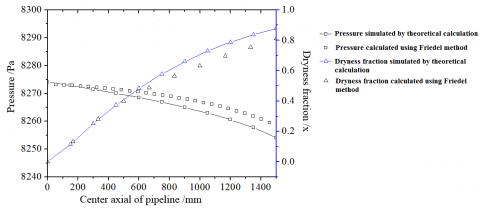Figure 2. Condensation model verification

2.3 Condensation model settings

The inlet fluid is a mixture of methane and water vapor and the boundary condition of inlet is inlet speed condition. The gas velocity is 14.44m/s and the mass fraction of saturated steam of 313.5 K is 0.056%. The Eulerian model is used to simulate gas-liquid two-phase flow. The boundary condition of outlet is free discharge and the sidewall is constant.

3. Results of Calculation and Analysis

3.1 Distribution of condensate

The simulation conditions are as follows at atmospheric pressure: the gravity is 9.81 m/s2, the inner diameter of pipe is 70 mm, the temperature of wall is 305 K, the temperature of inlet is 313.09 K, the gas flow is 200 m³/h and the gas velocity is 14.44 m/s. The simulation results of gas-liquid distribution at different elbow angles are shown in Fig.3. It shows that water vapor changes from uniform flow to non-uniform flow when flowing through the 60 ° elbow. The very high velocity zone appears in the inner side of the elbow and the velocity on the lower side of the pipe is greater than that on the upper side of the pipe after the elbow. For more detailed information of the flow field, we need to select three sections at the elbow perpendicular to the axis uniformly (i.e. section I, section II, section III) and another three sections at the straight pipe at intervals of 100mm after the elbow (i.e. section IV, section V, section VI), which are shown in Fig.3. Streamlines in the pipe of each section are shown in Fig.4. We can see from section I that the fluid flows from the lateral of the elbow to the medial of the elbow before entering the elbow, while flow field is uniform and there are no significant eddy currents. We can see from section II and III that vortices are generated after the fluid flows entering the elbow. The fluid flows along the sides of two vortices from the lateral of the elbow to the medial of the elbow, squeezing against the other and then flows from the medial to the lateral until it contacts the inner wall surface, finally diverting to both sides. By comparing section I, II, III and section IV, V, VI, we can see that vortices are generated after the fluid flows through the elbow and streamlines in the pipe at each section which has bilateral symmetry. As the fluid flows toward the outlet along the axial position, the vortices’ center gradually moves upward (toward the inside of the elbow).

## 3.png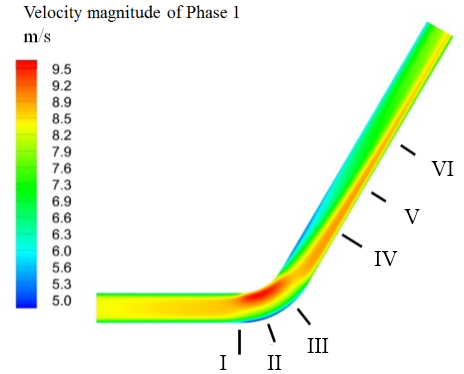Figure 3. Velocity contour

The phenomena observed from sections I, II, III, IV, V and VI conforms to the Boundary Layer Separation Theory in Fluid Mechanics. This theory refers to the fact that velocity gradient will change when viscous fluid is flowing through the surface causing the fluid in the boundary layer to stagnate and the boundary streamline to separate from the boundary  . This subsequently produces vortices . When fluid passes through the elbow, the changes in the direction of flow lead to two symmetrical vortex zones in the lateral and the medial of the elbow, which generates secondary circulation and causes loss of head to the elbow  .

The distribution of gaseous water (the mass ratio of water vapor to gas phase) in the 60° elbow section is analyzed and the results are shown in Fig. 5 and Fig. 6. The mass fraction of gaseous water near the wall of the elbow begins to decrease. In the straight pipe, the mass fraction of gaseous water near the wall becomes larger in the flow direction. The mass fraction of gaseous water in section IV is shown in the Fig. 6. The condensate is concentrated on the inside of the elbow, downstream of the straight pipe. In the flow direction, the concentrated distribution becomes more pronounced and eventually forms an inverted triangle condensate concentration area, which occupies from the upper part of the pipe to the center of the pipe. The region with little condensate gradually shifts towards the lower part (the outside of elbow) and distributes in the pipeline cheeks. The U-shaped non-condensing zone is formed, as seen in Section V and VI.

## 4.pngFigure 4. Streamline in pipe with 60°elbow

The relationship between the flow field and condensation distribution is analyzed by taking the inner cross section IV, V, VI of the straight section of the pipe with a 45° elbow angle. Then a comparative analysis of the cloud charts of velocity distribution (i.e. the three charts on the first line in Fig. 7) and mass fraction distribution (i.e. the three charts on the second line in Fig. 7) of gaseous water is carried out, as shown in Fig. 7.

Fig. 7 demonstrates a certain coincidence between the velocity distribution and the condensation distribution. In regions with greater velocity, the higher the mass fraction of gaseous water, the less the amount of condensate of water vapor. Conversely, in a region of lesser velocity there is low content of the gaseous water and a large amount of condensate of water vapor. In other words, the high velocity region is approximately coincident with the non-condensing region, while the low velocity region is approximately coincident with the condensation region. The results show that the velocity flow has a great influence on the condensation process.

## 5.png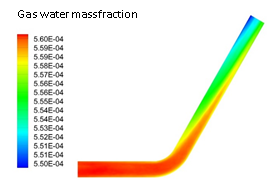Figure 5. Gaseous water mass fraction in the longitudinal section

## 6.png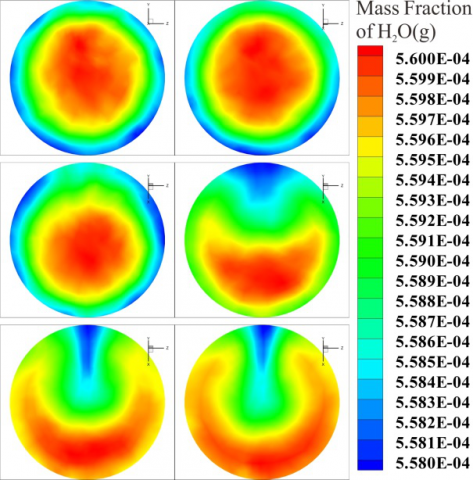Figure 6. Gaseous water mass fraction in different sections

## 7.png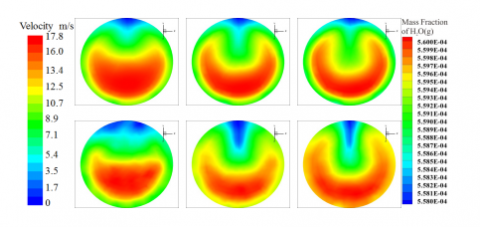Figure 7. Comparison of velocity and gaseous water mass fraction

3.2 Condensate

## 8.png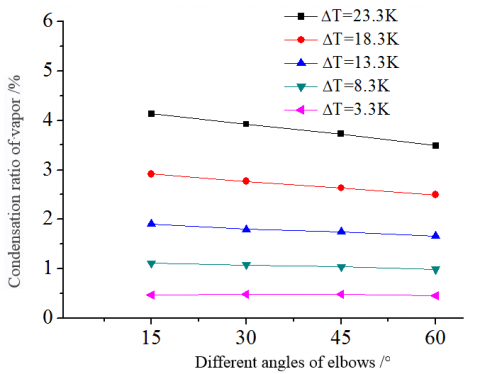Figure 8. Condensation with different elbow angles

## 9.png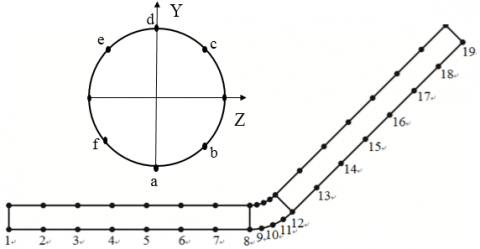Figure 9. Distribution of 6 Points and 19 Positions

The mass fraction differences between the inlet and outlet gaseous water, changes with the angles of elbows and the temperature differences between inner and outer surface, as shown in Fig. 8. It shows that with the increase of temperature differences between the inner and outer surface, the amount of condensation increases with the increase of elbow angle and the amount of condensation becomes smaller. This is due to the change of the elbow angle leading to varying degrees of turbulence, thus changing the convective heat-transfer coefficient so that the condensation in the pipe changes . In addition to this, the mixing ratio of water vapor and methane is also a factor affecting the heat-transfer coefficient , which is not discussed in detail here.

## 10.png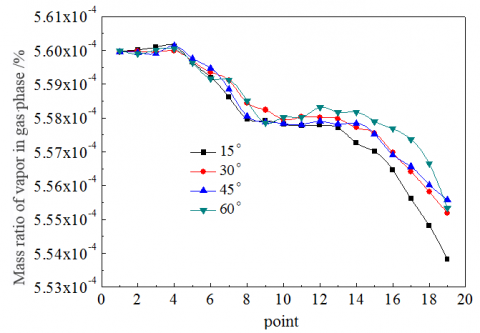Figure 10. Gaseous water quality in pipelines with different angles of Point a

## 11.png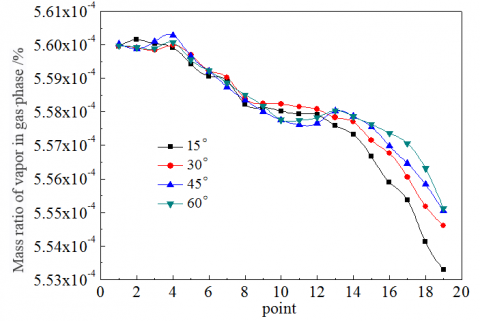Figure 11. Gaseous water quality in pipelines with different angles of Point b

## 12.png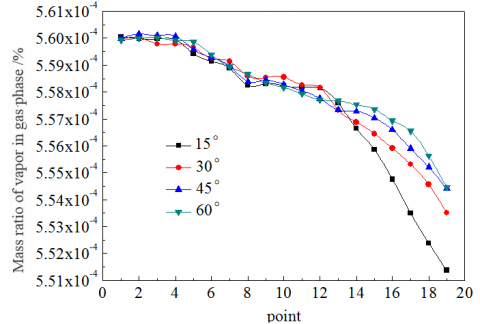Figure 12. Gaseous water quality in pipelines with different angles of Point c

## 13.pngFigure 13. Gaseous water quality in pipelines with different angles of Point d

## 14.png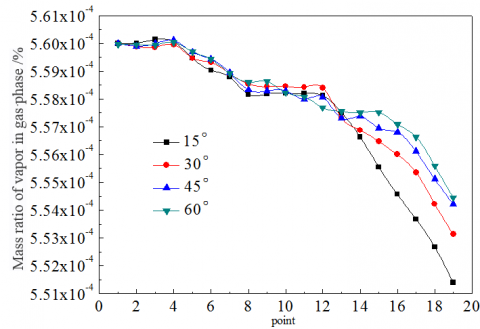Figure 14. Gaseous water quality in pipelines with different angles of Point e

## 15.png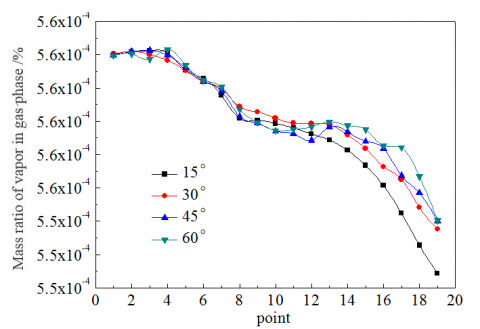Figure 15. Gaseous water quality in pipelines with different angles of Point f

In order to quantify the distribution of condensate near the wall and to reveal the potential condensing zone in the natural gas pipeline, six points (i.e. point a b c d e f) are taken in the radial direction of 1 mm from the tube wall evenly as the starting point and six curves parallel to the axis of the pipeline are plotted, each of them with 19 data points, as shown in Fig. 9. Data points closed to the wall of the straight pipe are labeled as Position 1-7. Data points at elbow are labeled as Position 8-12. Data points near the downstream of elbow are labeled as Position 13-19. The mass fraction of gas phase water of each pipe point with different elbow angles was analysed and is shown in Fig. 10-15. The result shows that the mass fraction of gaseous water on each straight line of the straight pipe decreases very slowly, especially in the straight pipe section before Position 5. The mass fraction of gaseous water closed to the wall remains basically unchanged. In addition, the mass fraction of gaseous water under different elbow angles is effectively the same. It is worth mentioning that, for the front straight section of the elbow (i.e. Position 5 to Position 8), the condensate in the pipe increases clearly. Combined with velocity streamlines in Fig. 4, the transverse vortex in the cross section of the front tube in the elbow is affected by downstream elbow which exacerbates convection in the pipe, subsequently enhancing the condensation of steam. There is no obvious change of condensate in the elbow (i.e. position 8 to position 12). The flow rate in the elbow is increased so that residence time of the fluid in the elbow is shortened, resulting in less heat transfer. Therefore, there is less condensate in the pipe. In the straight pipe after the elbow, the condensate in the pipe clearly increases. The mass fraction of gaseous water under different elbow angles was analyzed. The results indicate that the maximum and minimum condensated vapor are separately generated at 15° and 60° elbow.

4. Conclusions

The condensate in natural gas pipelines will aggravate the corrosion on the inner wall of pipelines. To address this problem, the early adoption of numerical simulation on the basis of theoretical analysis, was studied in this paper. The numerical calculation of condensation characteristics of low vapor content pipeline, leads to the following conclusions:

(1) The condensation model of gas pipelines of low vapor content, embedded in CFD calculation software is established which is then used to calculate the condensation process in elbows. The pressure variation during the condensing process is calculated by the Friedel method. The error between the numerical simulation and the theoretical calculation is less than 10%.

(2) When wet natural gas passes through the elbow, two symmetrical vortices are formed which generate secondary circulation and lead to non-uniform distribution of the flow field in the pipe. This phenomenon is more pronounced in the straight pipe after the elbow. The greater the deflection angle is, the more pronounced the non-uniform is.

(3) The condensation in the elbow is asymmetrical and the condensate on the inner side of the pipe elbow is more than that on the outside of the pipe elbow.

(4) Condensated liquid-phase distributions with four different elbow angles (i.e. 15°, 30°, 45° and 60°) were compared. The results show that the maximum and minimum condensate are separately generated at 15° and 60° elbow.

The gas-liquid two-phase information of the pipe wall obtained in the paper can provide theoretical references for reducing the corrosion of natural gas pipeline.

References

 López J., Pineda H., et al. (2016). Study of liquid–gas two-phase flow in horizontal pipes using high speed filming and computational fluid dynamics, Experimental Thermal and Fluid Science, Vol. 76, No. 9, pp. 126-134. DOI: 10.1016/j.expthermflusci.2016.02.013

 Svend T.M., Morten H. (2015). Depressurization of CO2-rich mixtures in pipes: Two-phase flow modelling and comparison with experiments, International Journal of Greenhouse Gas Control, Vol. 37, No. 6, pp. 398-411. DOI: 10.1016/j.ijggc.2015.03.029

 Swanand M.B., Afshin J.G. (2014). A flow pattern independent drift flux model based void fraction correlation for a wide range of gas–liquid two phase flow, International Journal of Multiphase Flow, Vol. 59, No. 2, pp. 186-205. DOI: 10.1016/j.ijmultiphaseflow.2013.11.001

 Guan X.R., et al. (2015). Numerical analysis of quasi-steady flow characteristics in large diameter pipes with low liquid loading under high pressure, Journal of Natural Gas Science and Engineering, Vol. 26, pp. 907-920. DOI: 10.1016/j.jngse.2015.07.039

 Van’t Westende, et al. (2007). Effect of secondary flow on droplet distribution and deposition in horizontal annular pipe flow, International Journal of Multiphase Flow, Vol. 33, pp. 67-85. DOI: 10.1016/j.ijmultiphaseflow.2006.07.004

 Li W.D., Li R.X., Wang Y.S., et al. (2001). Model for prediction of circumferential distribution of film thickness in horizontal gas-liquid annular flow, Chemical Industry and Engineering, Vol. 52, No. 3, pp. 204-208.

 Liang F.C., Cao X.W., Wang D., et al. (2008). A simplified model for circumferential film thickness distribution prediction of horizontal gas-liquid annular flow, Journal of Yangtze University (Natural Science Edition), Vol.5, No. 3, pp. 37-39,384.

 Hou H., Bi Q.C., Ma H. (2011). Conductance probe measurement method for falling film thickness around horizontal tube, Journal of Shenyang University of Technology, Vol. 33, No. 4, pp. 476-480.

 Andrea C., John R.T. (2013). Liquid film circumferential asymmetry prediction in horizontal annular two-phase flow, International Journal of Multiphase Flow, Vol. 51, pp. 44-54. DOI: 10.1016/j.ijmultiphaseflow.2012.12.003

 Li Y.X., Feng S.C. (2000). A study of the formation mechanism of annular flow liquid film and the flow pattern conversion, Natural Gas Industry, Vol. 20, No. 1, pp. 78-82.

 Banafi A., Talaei M.R., Ghafoori M.J. (2014). A comprehensive comparison of the performance of several popular models to predict pressure drop in stratified gas–liquid flow with low liquid loading, Journal of Natural Gas Science and Engineering, Vol. 21, No. 11, pp. 433-441. DOI: 10.1016/j.jngse.2014.09.009

 Banafi A., Talaie M.R. (2015). A new mechanistic model to predict gas–liquid interface shape of gas–liquid flow through pipes with low liquid loading, American Institute of Chemical Engineers, Vol. 21, No. 3, pp. 1043-1053. DOI: 10.1002/aic.14696

 Andrea C., Lorenzo S. (2016). Two-phase pressure drop prediction in helically coiled steam generators for nuclear power applications, International Journal of Heat and Mass Transfer, Vol. 100, No. 9, pp. 825-834. DOI: 10.1016/j.ijheatmasstransfer.2016.05.027

 Witkowski A., Majkut M. (2015) Analysis of Transportation Systems for CO2 sequestration, Advances in Carbon Dioxide Compression and Pipeline Transportation Processes. Springer, Cham, pp. 73-93.

 Blazek J. (2015). Boundary conditions, Computational Fluid Dynamics: Principles and Applications, Butterworth-Heinemann, pp. 253-282

 Joseph D.D., Renardy Y. (2013). Asymptotic analysis for long waves, Fundamentals of Two-Fluid Dynamics: Part I: Mathematical Theory and Applications, Springer Science, Business Media, pp. 279-290.

 Chang P.K. (2013). Morden development of flow separation theory, Separation of Flow, Elsevier, pp. 27-35.

 Thakare H.R., Monde A., Parekh A.D. (2015). Experimental, computational and optimization studies of temperature separation and flow physics of vortex tube: A review, Renewable and Sustainable Energy Reviews, Vol. 52, No. 7, pp. 1043-1071. DOI: 10.1016/j.rser.2015.07.198

 Wei L., Yong Li., et al. (2016). Experimental study of gas-liquid two-phase flow for high velocity in inclined medium size tube and verification of pressure calculation methods, International Journal of Heat and Technology, Vol. 34, No. 3, pp. 455-464. DOI: 10.18280/IJHT.340315

 Rafiee S.E., Sadeghiazad M.M. (2016). Heat and mass transfer between cold and hot vortex cores inside ranque-hilsch vortex tube-optimization of hot tube length, International Journal of Heat and Technology, Vol. 34, No. 1, pp. 31-38. DOI: 10.18280/IJHT.340105

 Barraza R., Nellis G., Klein S., et al. (2016). Measured and predicted heat transfer coefficients for boiling zeotropic mixed refrigerants in horizontal tubes, International Journal of Heat and Mass Transfer, Vol. 97, No. 2, pp. 683-695. DOI: 10.1016/j.ijheatmasstransfer.2016.02.030